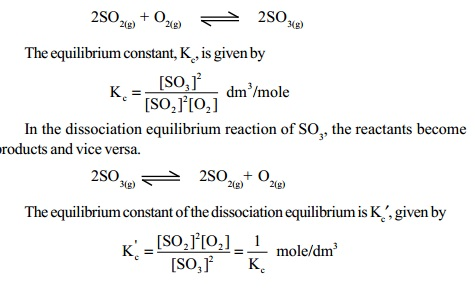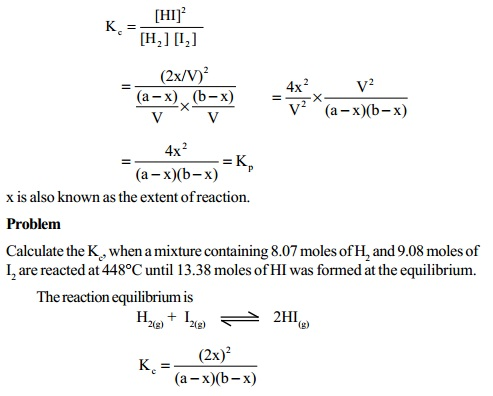Home | | Chemistry | Attainment Of Equilibrium In Chemical Reactions

# Attainment Of Equilibrium In Chemical Reactions

A chemical reaction in equilibrium involves the opposing reactions. One of the reactions produces the products and is known as the forward reaction while the other produces the reactants from products and is known as the reverse reaction.

ATTAINMENT OF EQUILIBRIUM IN CHEMICAL REACTIONS

A chemical reaction in equilibrium involves the opposing reactions. One of  the reactions produces the products and is known as the forward reaction while the other produces the reactants from products and is known as the reverse reaction. Chemical equilibrium is dynamic in nature. At equilibrium, reactant and product molecules are both present in the reaction mixture in definite amounts. The equilibrium concentrations of the reactants and products do not change under constant temperature, pressure and catalysts etc.

Consider a general equilibrium reaction at constant temperature represented  By

aA + bB -- kf  -- > < -- kr  -- cC + dD

According to law of mass action, the rate of forward reaction is

Rf = kf [A]a [B]b

and the rate of reverse reaction is

Rr = kr [C]c [D]d

where kf and kr are the rate constants of the forward and reverse reactions respectively.

At equilibrium Rf= Rr

kf [A]a [B]b = kr [C]c [D]d

k f / k r  = [C]c [D]d    / [A]a [B]b

kf / k r = Kc = equilibrium constant

Kc = [C]c [D]d    / [A]a [B]b

This equation of Kc is also known as equilibrium law equation. Kc is the equilibrium constant expressed in terms of molar concentrations of reactants and products. For reactions involving gaseous reactants or products or both, it is

more convenient to use partial pressures instead of molar concentrations. Thus Kc in equilibrium law equation becomes Kp which is the equilibrium constant expressed in terms of partial pressure.

1. Relationship between Kp and Kc

Consider a general chemical equilibrium reaction in which the reactants and products are in gaseous phases,

aA + bB + cC + ........    lL + mM + nN + .....Kp = Kc(RT) Dng

where Dng = total number of stoichiometric moles of gaseous products - total number of stoichiometric moles of gaseous reactants.

Usually, depending on the sign of Dng, Kp and Kc are related in three ways.

Case (i)

When Dng = 0, the total number of moles of gaseous products are equal to the total number of moles of gaseous reactants.

For example, in the formation equilibrium of HI,

H2(g) + I2(g) -- kf  -- > < -- kr  -- 2HI(g)

Dng = 2-(1+1) = 2-2      =0

Kp     = Kc (RT)O

Kp   = Kc

Case (ii)

When Dng = +ve, the total number of moles of gaseous products are greater  than the total number of moles of gaseous reactants.

For example,

2H2O(g) + 2Cl2(g) ) -- kf  -- > < -- kr  -- 4HCl(g) + O2(g)

Dng = (4+1) - (2+2)

= 5-4=1

Kp   = Kc (RT)1

Kp = Kc RT

and Kp> Kc

Case (iii)

When Dng = -ve, the total number of moles of gaseous products are lesser  than the total number of moles of gaseous reactants.

For example, consider the formation equilibrium of ammonia,

3H2(g) + N2(g) -- kf  -- > < -- kr  -- 2NH3(g)

Dng = 2 - (3+1)

= 2 - 4 = -2

Kp = Kc (RT)-2

Kp = Kc 2

(RT)

and Kp< Kc

2 Dependance of dissociation constant with formation equilibrium constant

In a formation equilibrium reaction, the reactants and products are written at the LHS and RHS of the equilibrium sign respectively. For the same reaction, the dissociation equilibrium consists of the products in the place of reactants and reactants in the place of products being written at the LHS and RHS of the equilibrium sign respectively.

In such cases, the equilibrium constant of the dissociation equilibrium reaction  which is also known as the dissociation constant, is found to be the reciprocal value of the equilibrium constant for the formation equilibrium reaction. For example, consider the formation equilibrium reaction of SO3, from SO2 and O2 gases,Kc′ is considered as the dissociation constant of SO3 gas. Usually, the equilibrium constant of the dissociation equilibrium is the reciprocal of the equilibrium constant of the formation equilibrium reaction.

3 Applications of law of chemical equilibrium to homogeneous equilibria

Formation equilibrium of HI

H2(g) + I2(g)  -- > < -- 2HI(g)

In this equilibrium reaction, the number of moles of the products is equal to the number of moles of the reactants (Dng = 0). Let us assume 'a' and 'b' moles of H2 and I2 gases being present in 'V' litres of the reaction vessel. At equilibrium, let x moles each of H2 and I2 react to form 2x moles of HI. Then, the equilibrium concentrations in moles litre of H2, I2 and HI in the reaction mixture will be (a-x)/V, (b-x)/V and 2x/V respectively. Since Dng = 0, Kc = Kp.Study Material, Lecturing Notes, Assignment, Reference, Wiki description explanation, brief detail
11th 12th std standard Class Organic Inorganic Physical Chemistry Higher secondary school College Notes : Attainment Of Equilibrium In Chemical Reactions |